# Intermediate maths solutions for Quadratic expressions second year

Intermediate mathematics second year textbook exercise solutions for Quadratic Expressions.

These solutions are very easy to understand. These are helpful for examination purpose.

Observe the given solutions and try them in your own method.

You can also see

Inter Maths 1A textbook solutions

Inter Maths 1B textbook solutions

Inter Maths IIA textbook solutions

Inter Maths IIB textbook solutions

Exercise 3(a)

Exercise 3(b)

Exercise 3(c)

M

## Intermediate 2a (IIA) chapter 3

Exercise 3(a)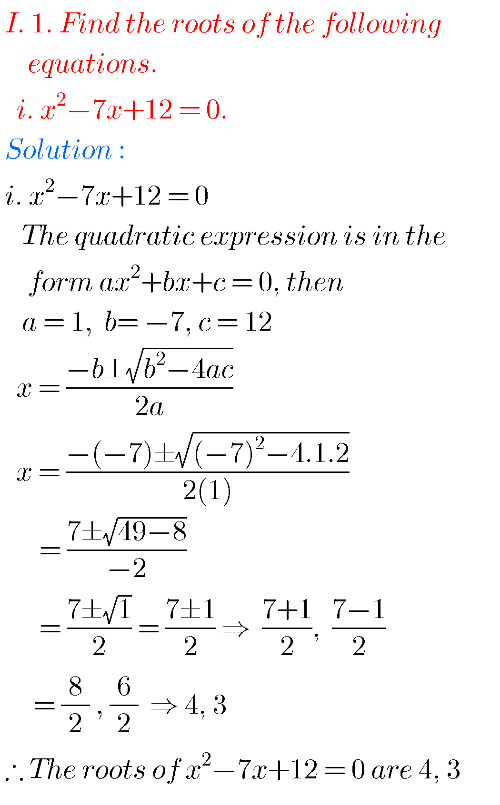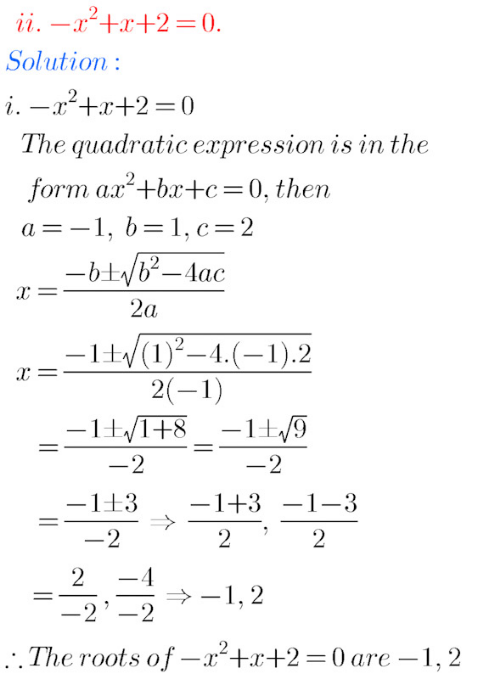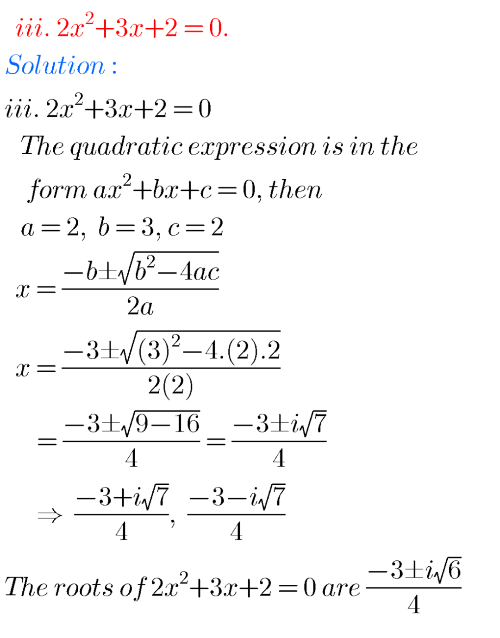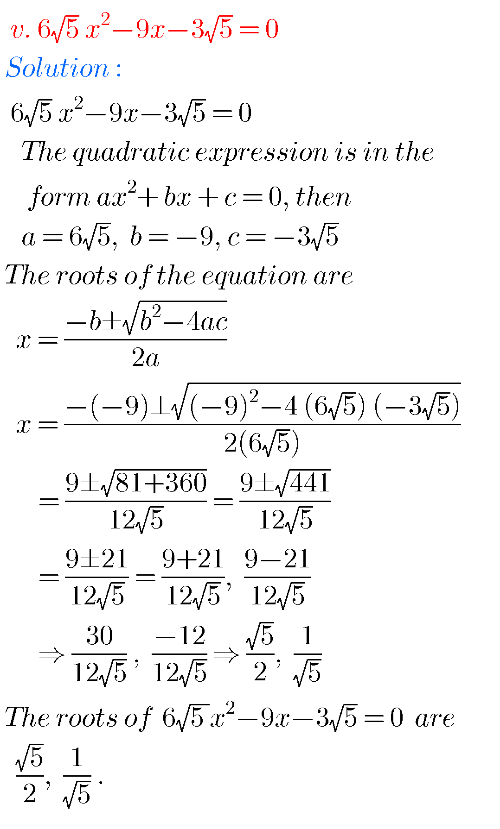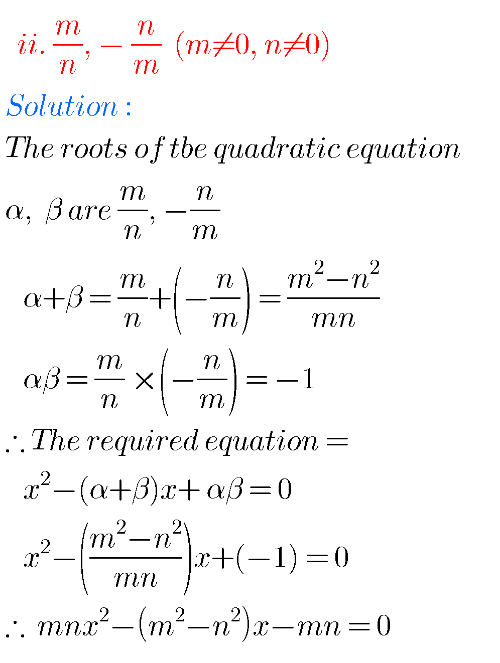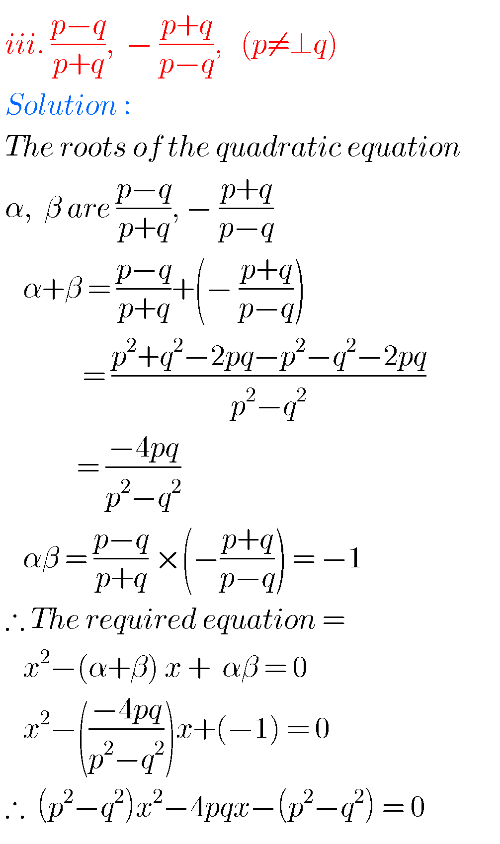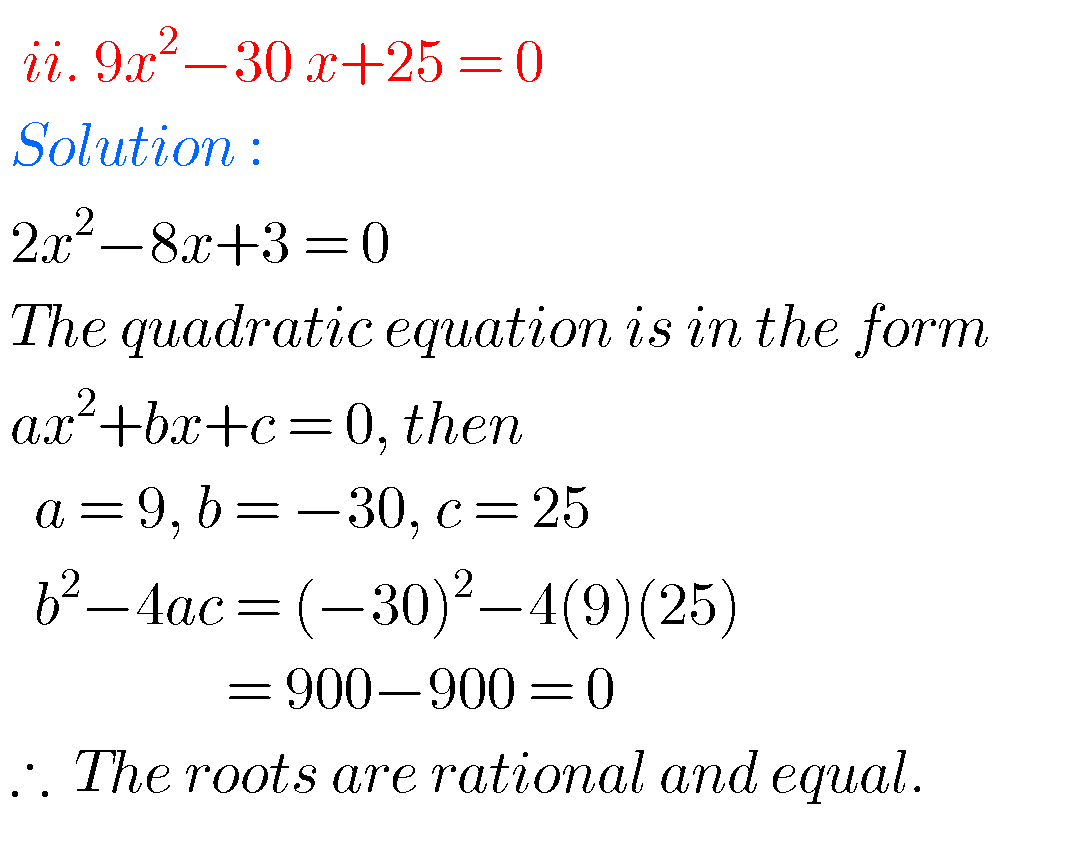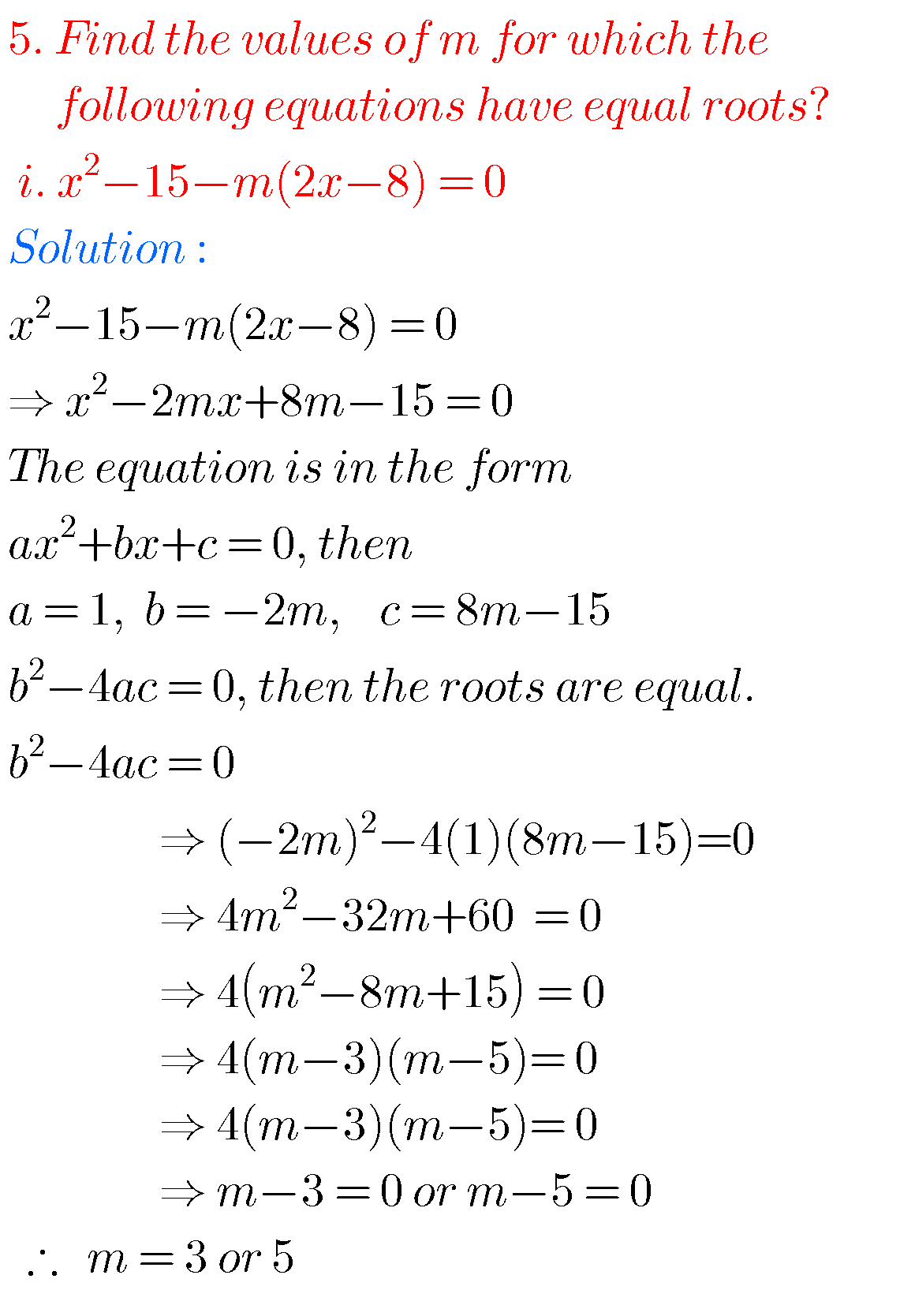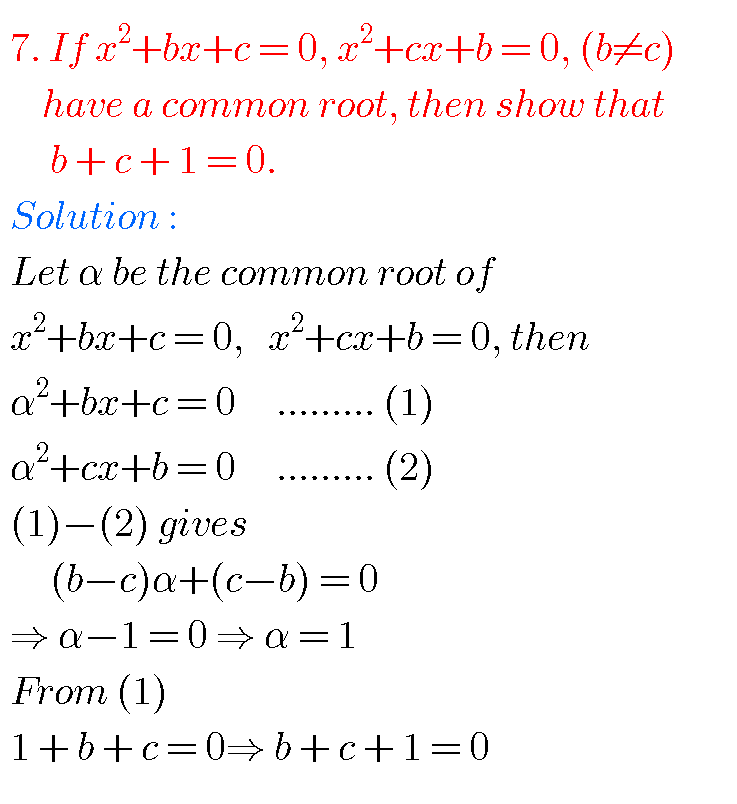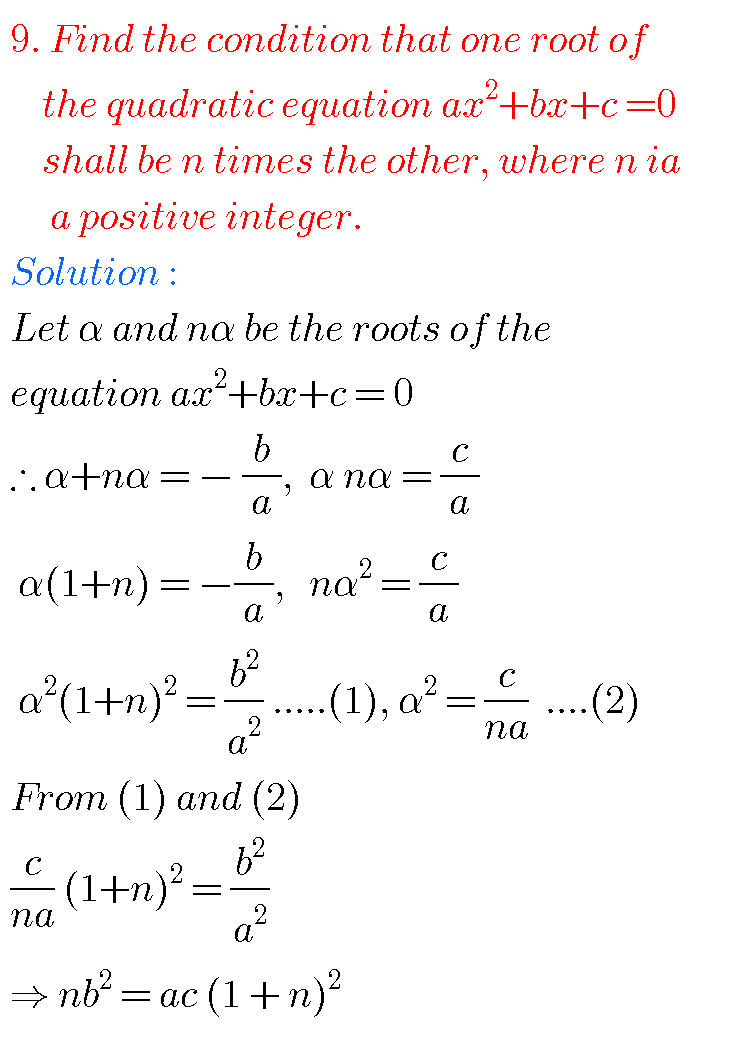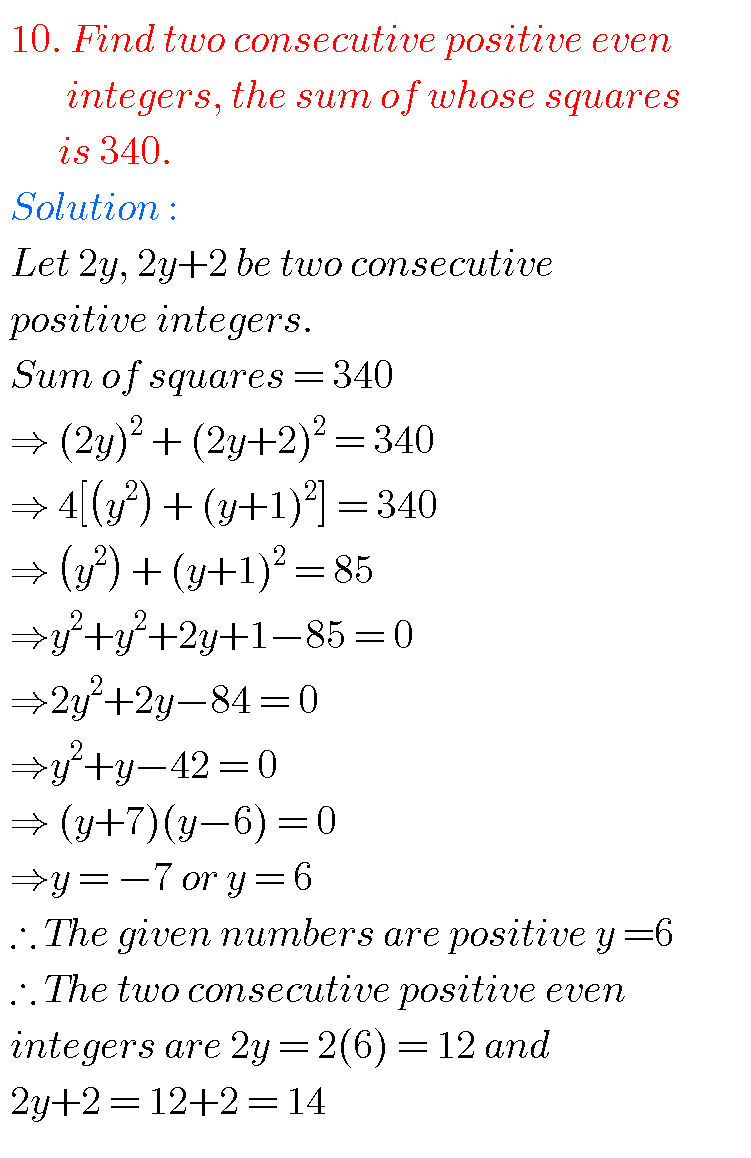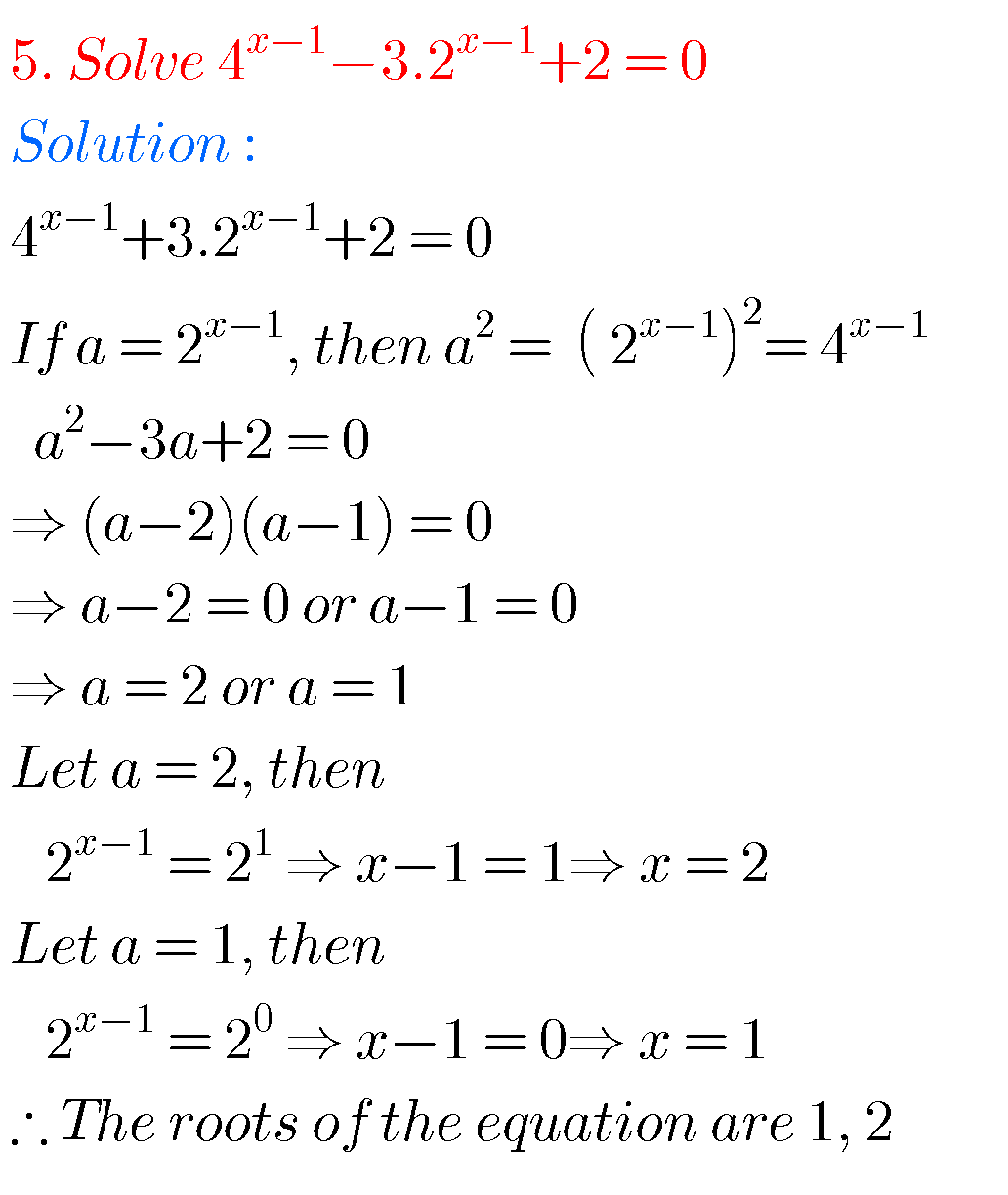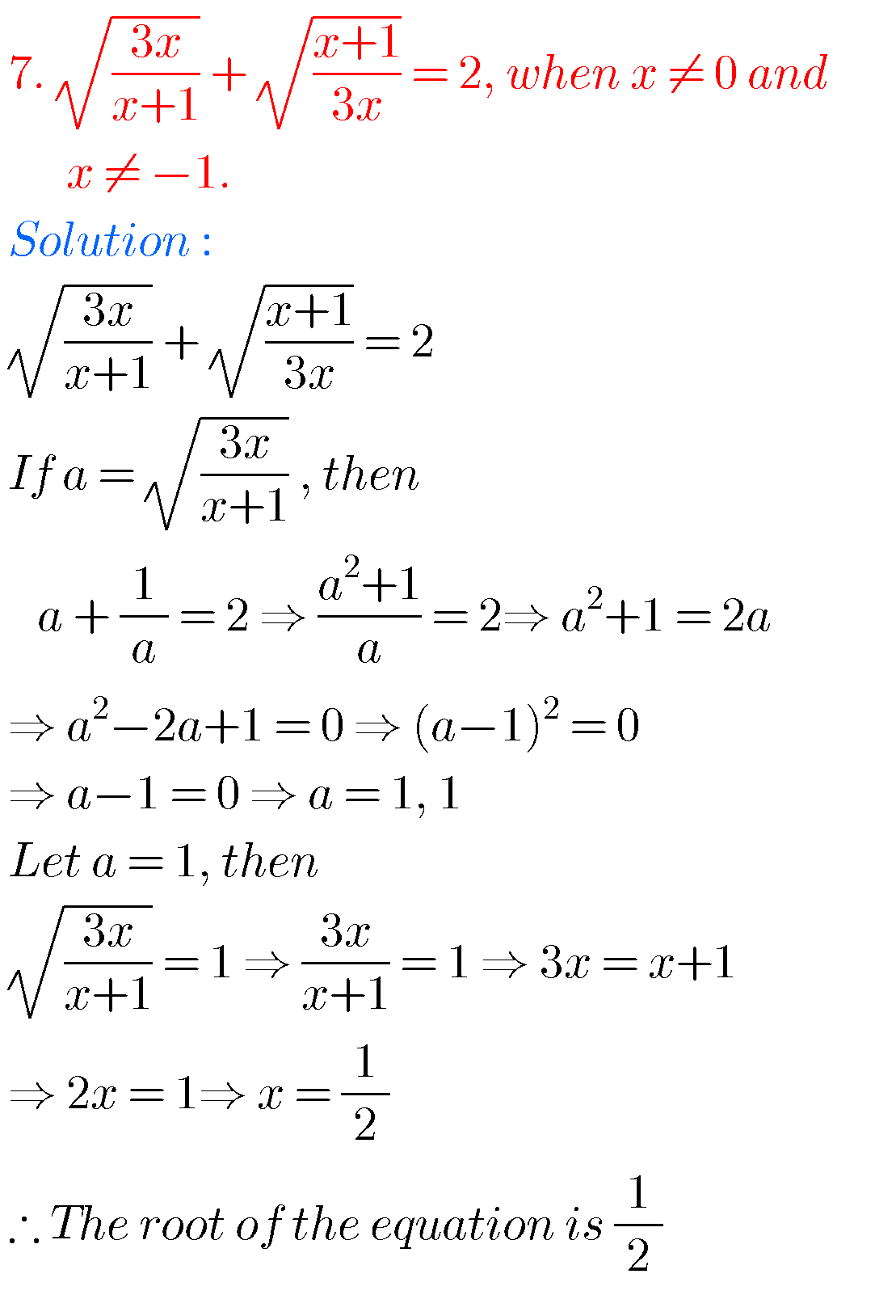### Mathematics inter quadratic expressions solutions

Some more solutions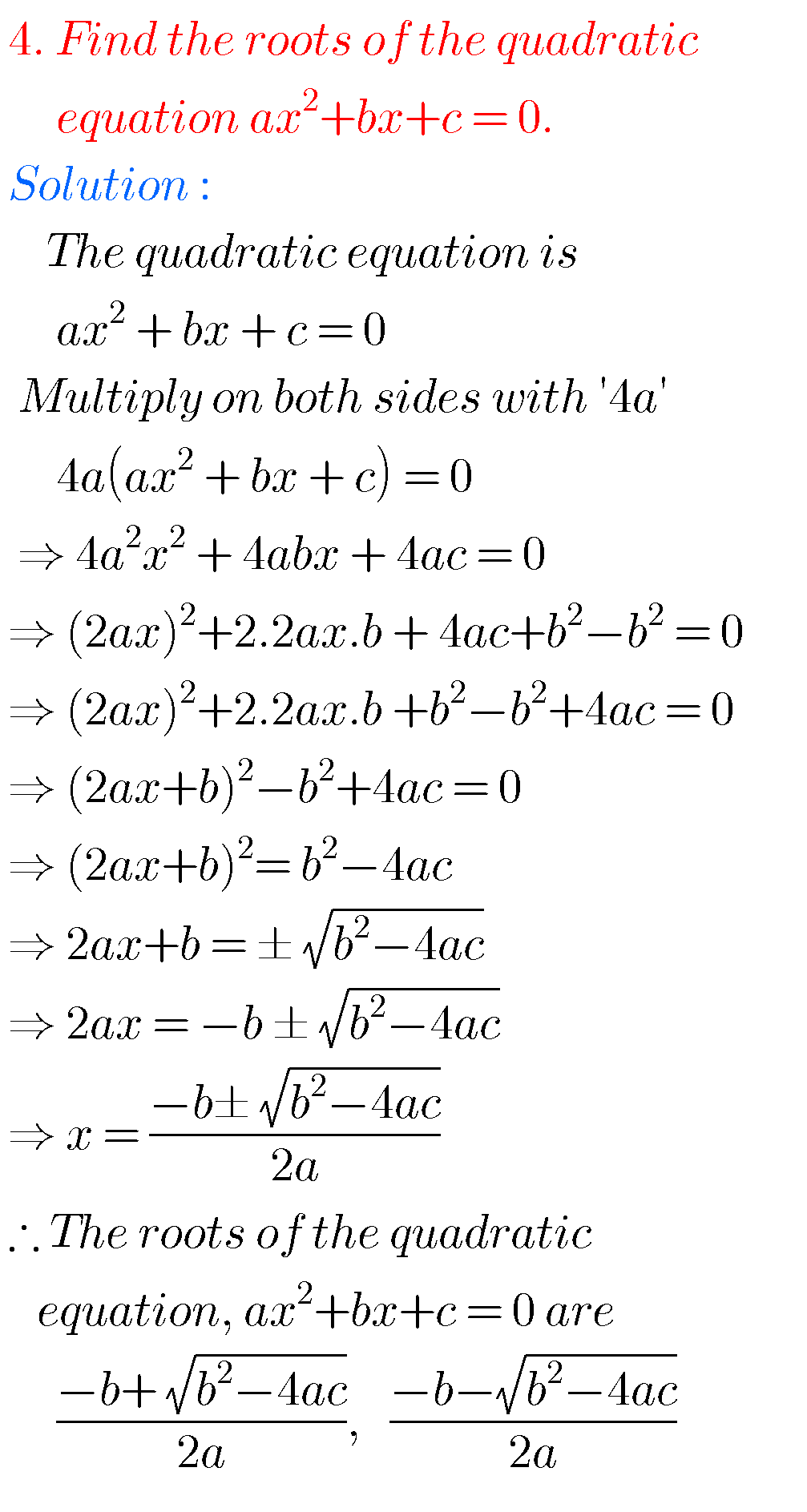###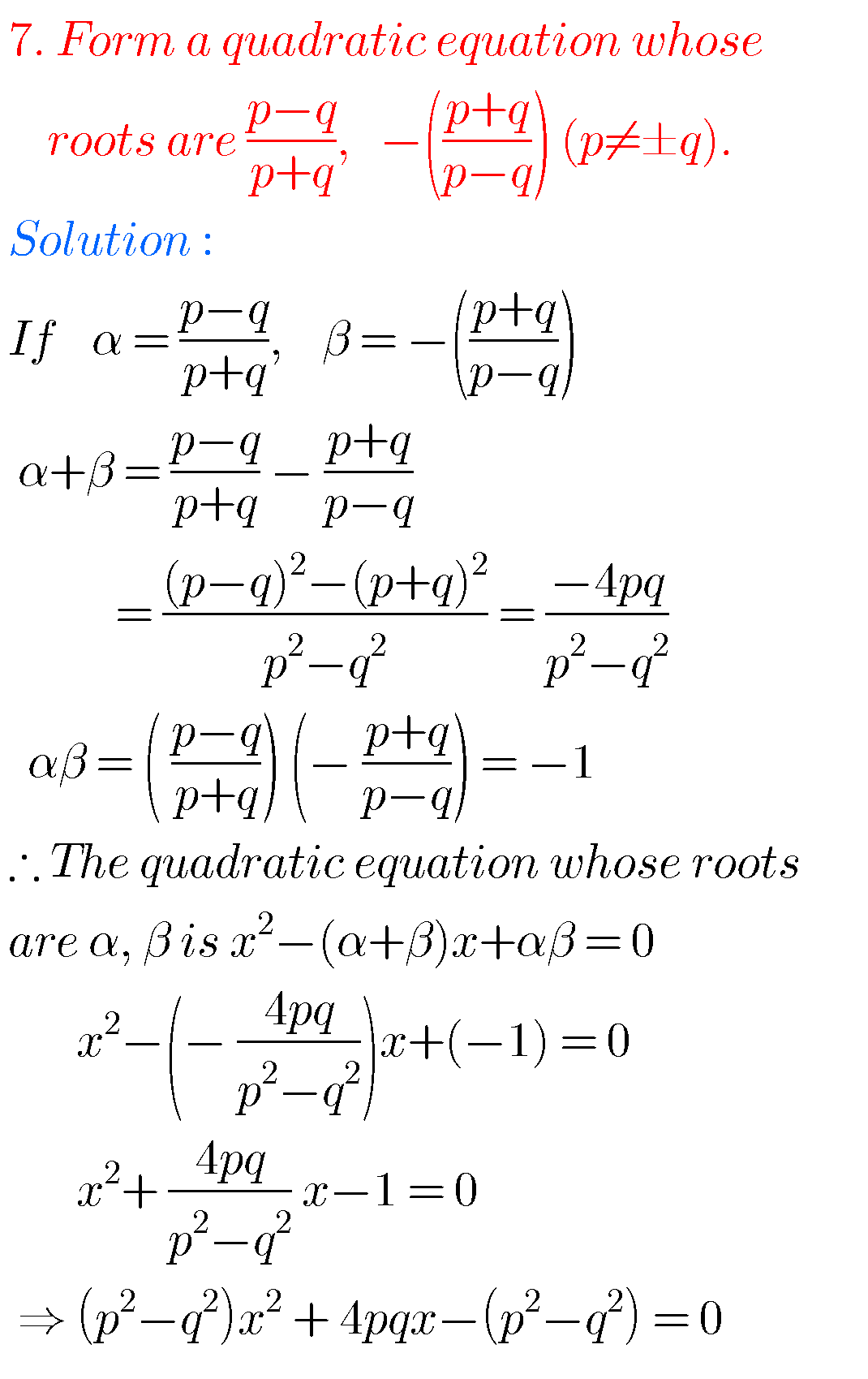###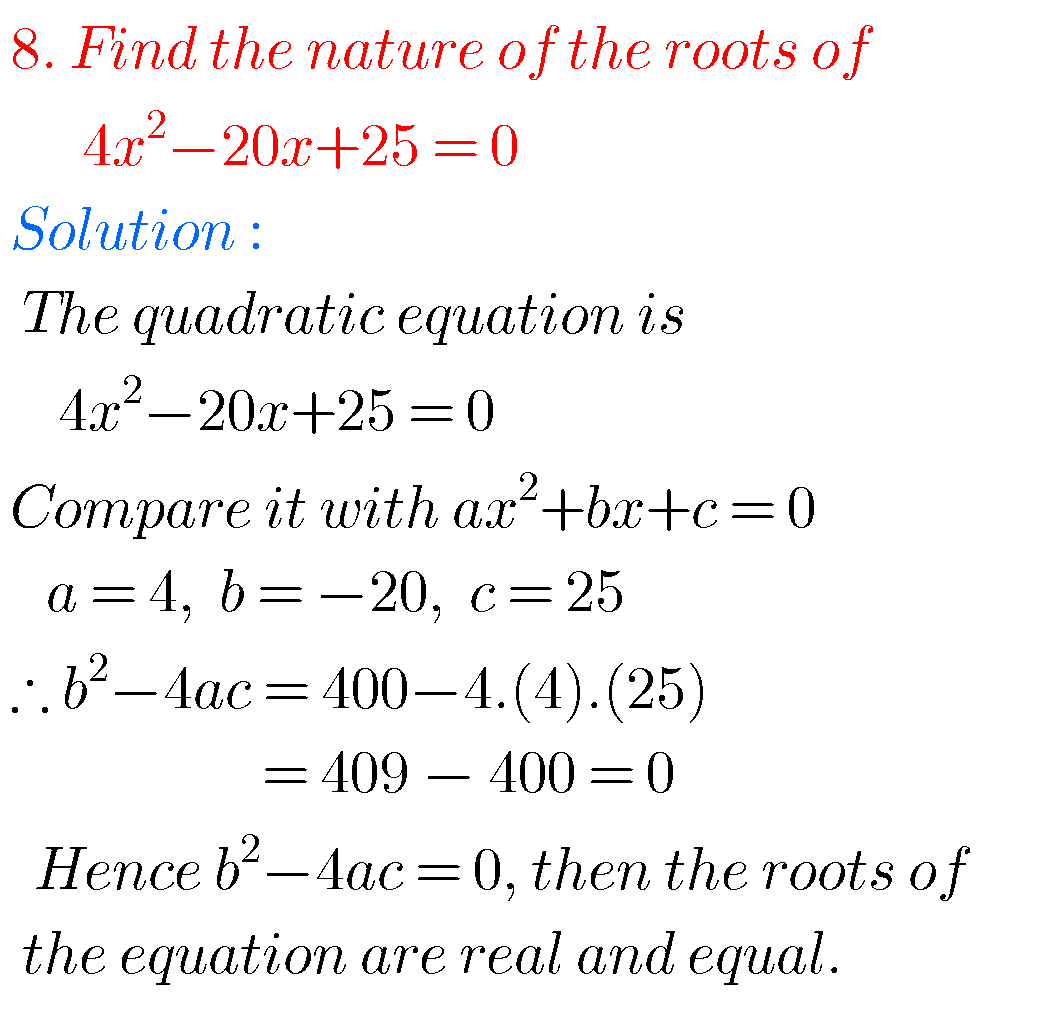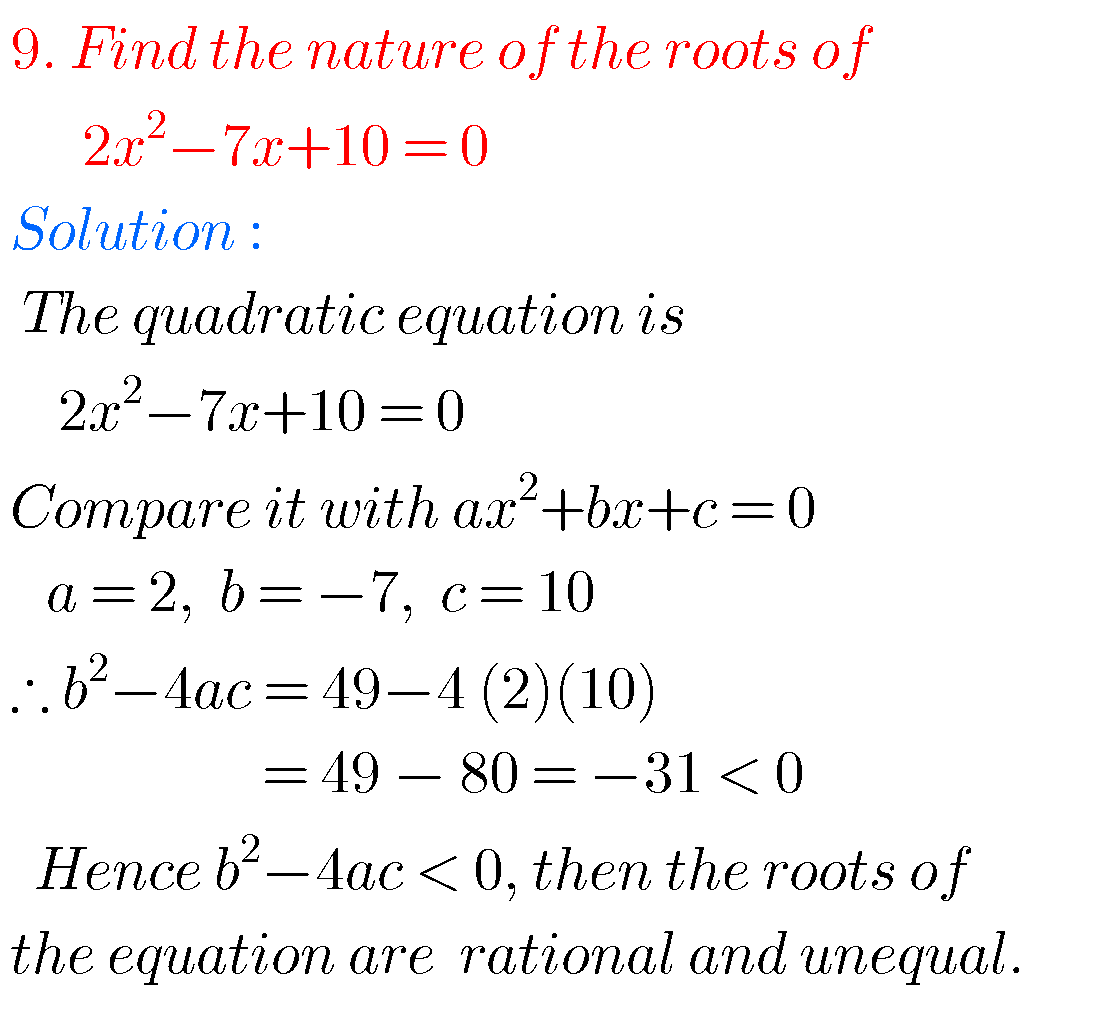Note : Observe the solutions and try them in your own methods.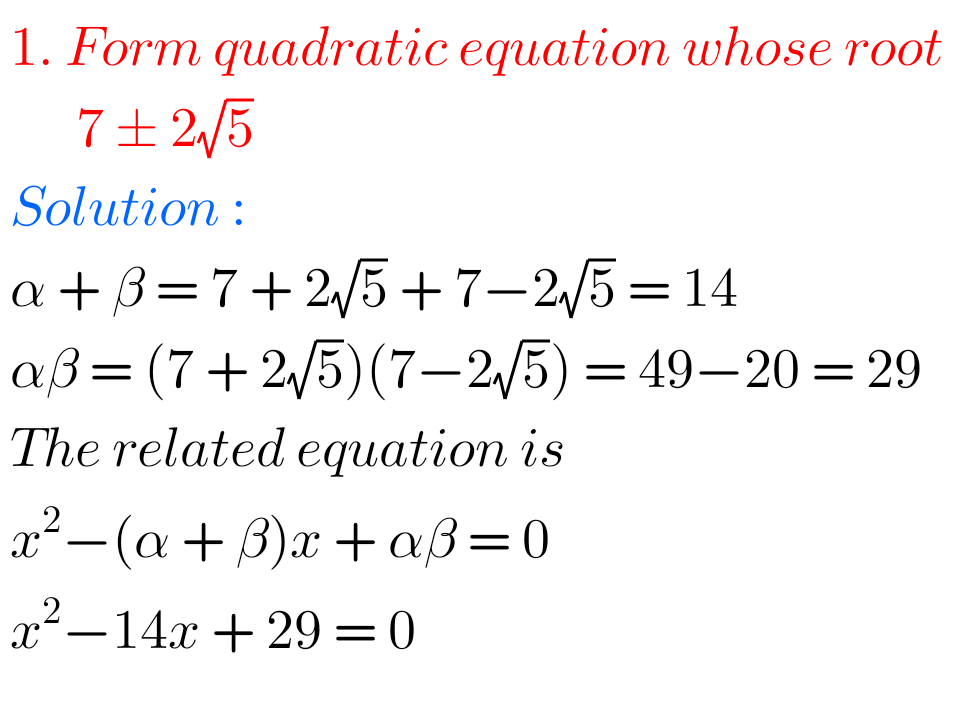Class 10 solutions for sets

Inter second year solutions for complex numbers

Inter second year solutions for De Moivre’ s theorem

Ncert solutions for class 8 exponents and powers

Exercise 1(b) inter maths 1A

### 4 thoughts on “Inter maths solutions for Quadratic Expressions second year,Intermediate Maths 2a(IIA) chapter 3 exercise 3(a) text book solutions”

1.Very nice S

2.Thank you

3.tq

4.Thank you so much